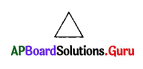Practice the AP 6th Class Maths Bits with Answers Chapter 9 2D-3D Shapes on a regular basis so that you can attempt exams with utmost confidence.

## AP State Syllabus 6th Class Maths Bits 9th Lesson 2D-3D Shapes with Answers

I. Choose the correct answer and write it in the brackets.

Question 1.
A 2-dimensional closed shape formed by straight lines is called ……………….
A) Plane
B) Space
C) Polygon
D) None
C) Polygon

Question 2.
Polygon means ……………..
A) Many sides
B) Two sides
C) One side
D) None
A) Many sidesQuestion 3.
Many sided figure is called ………………
A) Space
B) Polygon
C) Angle
D) Plane
B) Polygon

Question 4.
Four sided figure is called …………………
A) Triangle
B) Pentagon
C) Hexagon

Question 5.
Seven sided figure is called ……………….
A) Pentagon
B) Octagon
C) Heptagon
D) Nonagon
C) Heptagon

Question 6.
Ten sided figure is known as ………………
A) Decagon
B) Nonagon
C) Septagon
D) Hexagon
A) Decagon

Question 7.
Triangle has ……………….
A) Three sides
B) Three angles
C) Three vertices
D) All of these
D) All of these

Question 8.
Triangle can divides the points on the plane into …………… parts.
A) 4
B) 5
C) 3
D) 2
C) 3

Question 9.
The length of curved edge is called ………………
B) Circle
C) Circumference
D) Diameter
C) CircumferenceQuestion 10.
A chord which passes through the centre of the circle is called …………………
B) Diameter
C) Circle
D) Chord
B) Diameter

Question 11.
A line which divides the figure into two identical parts is known as ………………..
A) Line of symmetry
B) Line of straight
C) Line of diameter
D) Circumference
A) Line of symmetry

Question 12.
The shapes which have length, breadth and height (or) depth are called ……………….
A) 2D shapes
B) 4D shapes
C) 3D shapes
D) 5D shapes
C) 3D shapes

Question 13.
Example for the cuboidal shape
A) Triangle
B) Hexagon
C) Ball
D) Eraser
D) Eraser

Question 14.
If length, breadth and height are different, then die 3D-shape is known as
A) Cube
B) Cuboid
C) Cylinder
D) Prism
B) Cuboid

Question 15.
Example for a cylinder is ……………….
A) Pen
B) Candle
C) Pencil
D) All of the above
D) All of the above

Question 16.
Example for a sphere is ………………
A) Balls
C) Marbles
D) All of the above
D) All of the aboveQuestion 17.
Number of faces of a cube ……………..
A) 6
B) 8
C) 12
D) 3
A) 6

Question 18.
Cuboid has …………… edges.
A) 6
B) 8
C) 12
D) 3
C) 12

Question 19.
Vertices of a cube are ……………..
A) 6
B) 8
C) 12
D) 3
B) 8

Question 20.
Pyramid has …………… faces.
A) 5
B) 6
C) 8
D) 12
A) 5

Question 21.
Euler’s formula is ……………….
A) F + E = V + 2
B) F + V = E + 2
C) F + 2 = V + E
D) E + 2 = F + V
B) F + V = E + 2

II. Fill in the blanks.

1. Three sided figure is called …………………
Triangle

2. Five sided figure is called ………………
Pentagon

3. Six sided figure is called ……………….
Hexagon4. The least number of sides needed to form a polygon is ………………..
Three

5. The simplest closed figure formed by three line segments is called a ………………….
Triangle

6. A triangle is denoted by the symbol …………………..7. Locus of the points which is at equidistant from a fixed paint is called ……………….
Circle

8. The distance from the centre to any point on the circumference of circle is called ……………

9. Middle point of the diameter is known as ………………
Centre

10. A line segment joining two points on the circumference of the circle is called ………………….
Chord

11. The part of a circle between the two points is …………………
Arc

12. The region in the interior of a circle enclosed by the boundary is …………………
Circular region13. Another name of line of symmetry is …………………
Axis of symmetry

14. The shapes which have only length and breadth are known as …………………
2D shapes

15. The 3D shape in which has length, breadth and height are equal is …………………
Cube

16. Example for cone shape …………………
Joker cap/ Heap of grains

17. Example for pyramid shape ………………….
Egypt Mummy

18. The shape of dice is …………………
Cube

19. No. of edges of square pyramid …………………
820. Edges of a sphere ………………..
0 (No edges)

III. Match the following:

A)
Number of sides
1) 10
2) 3
3) 6
4) 9
5) 4
6) 5

Name of the polygon
b) Nonagon
c) Triangle
d) Decagon
e) Hexagon
f) Pentagon
1) 10 – d) Decagon
2) 3 – c) Triangle
3) 6 – e) Hexagon
4) 9 – b) Nonagon
6) 5 – f) Pentagon

B)
1) Cube
2) Cylinder
3) Square pyramid
4) Cone
5) Sphere
6) Rectangular prism

Number of edges
a) 12
b) 8
c) 1
d) 0
e) 9
f) 2
1) Cube – a) 12
2) Cylinder – f) 2
3) Square pyramid – b) 8
4) Cone – c) 1
5) Sphere – d) 0
6) Rectangular prism – a) 12C)
1) Cube
2) Pyramid
3) Cone
4) Cylinder
5) Triangle
6) Heptagon

Number of vertices
a) 1
b) 0
c) 5
d) 7
e) 8
f) 3